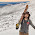Saturday, November 10, 2012

Physics of a Slapshot

By Dave Rappeport
With the hope that the 2012 NHL lockout may finally be coming to an end and the coming approach of winter, I decided I should deepen my understanding of the physics behind the game. More specifically, I want to understand the physics behind the slapshot. Zdeno Chara of the Boston Bruins currently holds the record for fastest slapshot at 108.8 mph, (48.6 m/s). How fast must Chara’s stick rotate for the puck to have that speed? How much does he bow his stick?

http://www.youtube.com/watch?v=bcieIx6_ArQ   (2 minute mark)

To simplify the problem:
-No friction
-No loss of velocity to bowing the stick

The physics of the slapshot can be summed up as an elastic collision whereby the kinetic energy in a hockey stick and the momentum of the stick are transferred to the puck.

The official weight of an NHL puck is 6 oz, (0.17 kg). If we assume the puck starts at rest and the stick is only in contact with the puck for 0.02s, we can use impulse to find the force exerted on the puck.

SΔFt = Δp
SΔF(0.02s) = 0.17kg(48.6 m/s -0)

SFx = 413 N
Wnet = SFx

The ΔKE is made up of two parts: rotational and translational kinetic energy. In order to calculate rotational energy, let’s model the stick as a long uniform rod rotating through its end. Therefore, the radius is equal to the length. The average professional defensemen’s stick is approximately 2.0 m in length.

How fast is Chara’s stick rotating just before it hits the puck?

I= (1/3)ML^2
L = 2.0m = r
v = rω
vi = 0 = ωi

Wnet = 413 N
Wnet = ΔKE

413 N = (1/2)M*(vf^2-vi^2) + (1/2)*(1/3)ML^2*(ωf^2-ωi^2)
= (1/2)M*(rωf^2) + (1/2)*(1/3)ML^2*(ωf^2)
= .5(1.0)(4)*(ωf^2) + (.17)(1.0)(4) *(ωf^2)
= 2.68*(ωf^2)

ωf = 12.4 rad/s

How much did Chara bow his stick?

First, we need the change in the spring potential energy stored in the stick when the player bends (bows) it. Hockey sticks have varying degrees of flex, the amount of force it takes to bend the stick one inch. In the context of spring force, flex rating = k.

If we assume Chara’s stick has a flex rating of 100 lbs, then k = -(440N/in), (11.2 N/m).

Now we need the change in gravitational potential energy. In order to find it, we need the height of the end of the stick during the player’s windup. 4Now let’s assume that the stick is not rotated a full 180° (π) but about 75% of 180° (π), (3π/4). The height of the stick would be given by the change in “l”, which = (3π/4)*(2.0m) = 4.7m.
Wnet = SFx
Wnet = 413 N
Wnet = ΔKE = -ΔPE

I= (1/3)ML^2
k = -(440N/in)
x = ?
h = 4.7m

413 N = Mgh + kx
= (1.0)(9.8)(4.7) + (-440)(x)

x = -.84 inches

1.Good equation to solve this

2.You can't equate a force to a change in energy... 401J of KE so if force is avg 413N then contact occurs for almost 1 m... Not for a slapshot! Also need to use a k in N/m units, which would be 15000... Unreasonable answers follow because you can't model this as elastic and get reasonable results.

1.Agreed. There are a number of problems in this post, which is likely true with a number of these posts. Students put them together and post them on their own (or send them to me and I post them without looking over them). Corrections to posts would be wonderful:)!

Note: Only a member of this blog may post a comment.